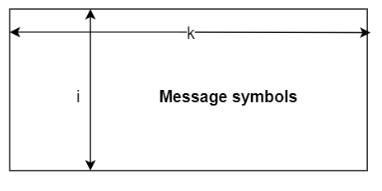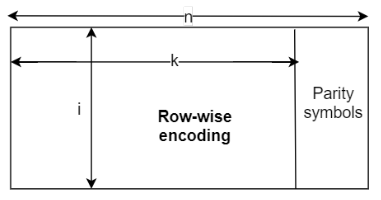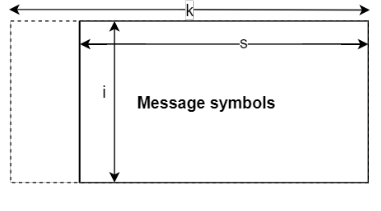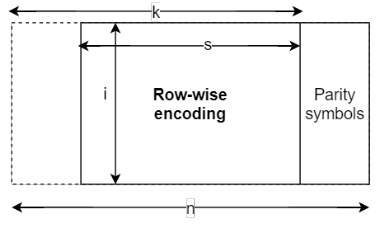ccsdsRSEncode

Encode CCSDS-compliant RS codes

Description

code = ccsdsRSEncode(msg,k) encodes the message in msg by using a (255, k) Reed-Solomon (RS) encoder, as defined in Consultative Committee for Space Data Systems (CCSDS) 131.0-B-3 Section 4 . k is the message length. code is in dual basis form, as the function assumes that the input to the CCSDS RS encoder is in dual basis form. For more details on dual basis representation, see CCSDS 131.0-B-3 Section 4.4.2 .

For a description of CCSDS RS code construction, see CCSDS RS Code Construction.

example

code = ccsdsRSEncode(msg,k,i) specifies the interleaving depth, i. msg consists of i RS message symbols of length k.

example

code = ccsdsRSEncode(msg,k,i,s) encodes the shortened input message of length s with interleaving depth i.

Examples

collapse all

Encode a message using a Consultative Committee for Space Data Systems (CCSDS) Reed-Solomon (RS) encoder.

Specify the message length, k, and the interleaving depth, i.

k = 239;
i = 3;

Generate a column vector of random message symbols. The length of the message is product of message length, k, and interleaving depth, i.

msg = randi([0 255],k*i,1);
size(msg)
ans = 1×2

717     1

Encode the message by using CCSDS RS encoder.

code = ccsdsRSEncode(msg,k,i);

Verify that the length of the encoded codeword is 255 times the value of the interleaving depth.

size(code)
ans = 1×2

765     1

Encode a message using a Consultative Committee for Space Data Systems (CCSDS) Reed-Solomon (RS) encoder with message shortening.

Specify the message length, k, interleaving depth, i, and the shortened message length, s.

k = 223;
i = 2;
s = 146;

Generate a column vector of random message bits. The length for the shortened message bits is eight times the product of shortened message length, s, and the interleaving depth, i.

msg = logical(randi([0 1],s*i*8,1));

Encode the shortened message by using a CCSDS RS encoder.

code = ccsdsRSEncode(msg,k,i,s);

Verify that the length of the encoded codeword is equal to (8*i*(255 – k + s).

size(code)
ans = 1×2

2848           1

Input Arguments

collapse all

Input message, specified as a column vector of logical bits or a column vector of integers in the range [0, 255]. The size of the column vector depends on the data type of the input message.

Input Message TypeSize of msg
Data Type of msg Is logicalData Type of msg Is uint8 or double
Full-length input message8*k k
Interleaved input message8*k*i k*i
Shortened input message8*s*i s*i

Data Types: double | uint8 | logical

Message length, specified as 223 or 239.

Data Types: double

Interleaving depth, specified as 1, 2, 3, 4, 5, or 8. The default value, 1, corresponds to no interleaving.

msg consists of i RS message symbols of length k.

Data Types: double

Shortened message length, specified as an integer in the range [1, k].

Data Types: double

Output Arguments

collapse all

CCSDS RS encoded message, returned as a column vector. The data type of code is same as that of the input message, msg. The size of the column vector depends on the data type of the input message.

Input Message TypeSize of code
Data Type of msg Is logicalData Type of msg Is uint8 or double
Full length input message8*255 255
Interleaved input message8*255*i 255*i
Shortened input message8*i*(255 – k + s)i*(255 – k + s)

collapse all

CCSDS RS Code Construction

CCSDS RS codes are powerful burst error-correcting codes used as forward error-correcting (FEC) codes.

The CCSDS RS encoder accepts full-length or shortened messages.

Construction of Full-Length Message CCSDS RS Codes

For full-length input messages the input column vector length is a product of the interleaving depth (i) and the message length (k).Encoding in CCSDS RS codes is done row-wise. The encoding results in an i-by-n vector that includes parity bits added to the end of each row. n is the codeword length, which is fixed to 255 symbols according to CCSDS 131.0-B-3 Section 4 .Construction of Shortened Message CCSDS RS Codes

For shortened input messages, the input column vector length is a product of the interleaving depth (i) and the shortened message length (s). The shortened message vector prepends padding the beginning of the message vector with zeros. The resulting vector is an i-by-k vector.Encoding in CCSDS RS codes is done row-wise. The encoding results in an i-by-n vector that includes parity bits added to the end of each row.TM Synchronization and Channel Coding. Recommendation for Space Data System Standards. CCSDS 131.0-B-3. Blue Book. Issue 3. Washington, D.C.: CCSDS, September 2017.

Satellite Communications Toolbox Documentation